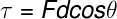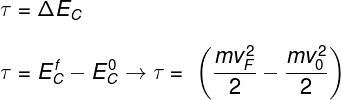Mechanics

# Energy

Energy is a physical quantity that is conserved, that is, the total amount of energy never changes. In different terms, energy is understood to be the ability to do work . There are several forms of energy interchangeable with each other, that is, they can be transformed into each other. Nevertheless, when transformed, energy can suffer degradations due to entropy , becoming less useful with each transformation.

In the context of physics in high school, some forms of energy are more studied than others, such as:

• Kinetic energy
• mechanical energy
• gravitational potential energy
• elastic potential energy
• Thermal energy
• Electricity
• Nuclear energy

## What is energy for physics?

The meaning of energy in physics is quite abstract: it is a quantity that is always conserved, that is, it never changes , regardless of the phenomenon studied.

In order for a body to do work or even change its temperature , it is necessary for some body to transfer part of its own energy to it. This energy transferred between bodies can undergo transformations and, therefore, can be expressed in many ways: potential, kinetic, thermal, electrical, chemical, nuclear and others.

According to the SI , the unit of measurement for energy is joule (J). By definition, 1 joule is the amount of energy that needs to be transferred for an object to move one meter against the action of an external force of 1 N. In addition to the joule, there are other units of energy measurement, such as the calorie.

Calorie is the energy required to heat 1 g of water by 1 °C under normal pressure conditions (1 atm ) . The quantitative relationship between the joule and calorie units was measured for the first time by the physicist James Prescott Joule (1818-1889). According to Joule’s findings, the mechanical equivalence of heat is such that 1 joule is equal to 4.1 cal .

As mentioned, a body endowed with energy is capable of performing work, that is, it has the ability to produce motion against the action of some external force . An example of this is when we move against the force of gravity — in doing so, we acquire gravitational potential energy. This acquired energy, however, was not created out of thin air: it was transformed, since, in order to move against gravity, our body consumed a certain amount of energy in the process. Understand, below, what is the relationship between work and energy.

forms of energy

There are many forms of energy, so in this topic we will list the most important ones and describe their main characteristics, check them out:

• Kinetic Energy: Every moving body has kinetic energy. This form of energy depends on the square of the speed with which the body moves and is proportional to its mass.
• Mechanical energy: is defined as the sum of kinetic energy and all forms of potential energy of a physical system. When there are no dissipative forces , mechanical energy is conserved .
• Gravitational potential energy: when a body is positioned at some height in relation to the ground, it has gravitational potential energy. This form of energy is related to mass, gravity and the height of the body in relation to the ground.
• Elastic potential energy: every body that tends to return to its original shape after being deformed has an amount of elastic potential energy. This energy depends on the square of the deformation of the body.
• Electrical energy: is the popular name used to designate electrical potential energy. The attraction between charges gives rise to it. This energy depends on the product between the charges and is inversely proportional to the distance that separates them.
• Thermal energy: is the sum of the kinetic energy of the particles of a body. This energy is directly related to the absolute temperature of the body, measured in kelvin. Also, the transfer of thermal energy between bodies is called heat .
• Nuclear energy: comes from the attractive forces that hold the atomic nucleus together. When the nucleus of atoms is disintegrated, it emits energy in the form of corpuscular and wave radiation .

If you are more curious about the topic of this topic, read our text: Forms of energy .

## Relationship between work and energy

Work and energy are quantities of the same dimension , that is, both are measured in joules. The work can be calculated by the inner product of the force and displacement vectors . Therefore, the component of force that is parallel to the direction of the distance traveled by the body contributes to the performance of work, while the perpendicular component does not promote any performance of work.

In other words, the part of the force that points in the direction of displacement of the body promotes the transfer of energy to that body. The figure below shows the formula used to calculate the work done by the application of force , check it out:F – force (N)

d – distance (m)

θ – angle between force and work (º)

In addition to the above definition, we know that the performance of work on a body promotes a change in kinetic energy. This variation is determined by the work and kinetic energy theorem , shown below:F e E i – Final and initial kinetic energies

According to this theorem, the performance of work is equivalent to the change in kinetic energy , calculated by the difference between the final and initial kinetic energy .

## energy conservation

As stated, energy is a quantity that is conserved, that is, the total amount of energy in a closed system is kept constant , however, it is also true that energy undergoes transformations and begins to express itself in other ways.

Imagine a system where a skateboard is set to oscillate on a concave-shaped track. Over time, the movement of the skateboard will cease, as all the kinetic energy and gravitational potential associated with it is gradually converted into thermal energy, thanks to the action of frictional forces between the bearings and also between the wheels of the skateboard and the floor.

Even though the mechanical energy of the skateboard was reduced, the total energy associated with it was still kept constant: if we added up the entire amount of thermal energy produced during its oscillation, we would find that there is no “energy loss” .

Although there was no loss, we say that the mechanical energy that has been transformed into thermal energy is dissipated energy, but we say that because, after being transformed into thermal energy, the mechanical energy cannot be reverted to its nature. original, at least not entirely. It’s as if the energy has lost quality and is now not as useful as it used to be. The phenomenon that explains energy degradation is known as entropy .

Thanks to the entropy phenomenon, described by the 2nd law of thermodynamics , it is not possible for any physical system to operate indefinitely . According to this premise, no machine can achieve 100% efficiency . In other words, the perpetual motor , also known as the perpetual motor, does not exist.